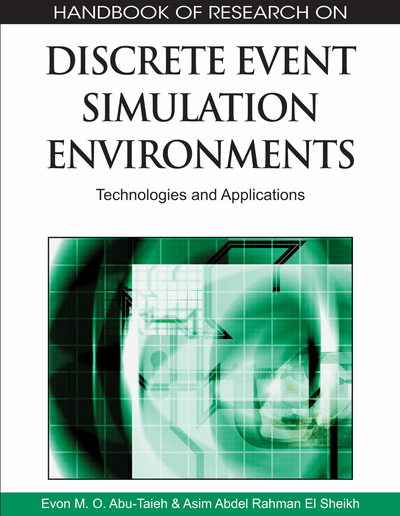# Experimental Error Measurement in Monte Carlo Simulation

Lucia Cassettari, Roberto Mosca, Roberto Revetria
DOI: 10.4018/978-1-60566-774-4.ch006
OnDemand:
(Individual Chapters)
Available
\$37.50
No Current Special Offers

## Abstract

This chapter describes the set up step series, developed by the Genoa Research Group on Production System Simulation at the beginning of the ’80s, as a sequence, through which it is possible at first statistically validate the simulator, then estimate the variables which effectively affect the different target functions, then obtain, through the regression meta-models, the relations linking the independent variables to the dependent ones (target functions) and, finally, proceed to the detection of the optimal functioning conditions. The authors pay great attention to the treatment, the evaluation and control of the Experimental Error, under the form of Mean Square Pure Error (MSPE), a measurement which is always culpably neglected in the traditional experimentation on the simulation models but, that potentially can consistently invalidate with its magnitude the value of the results obtained from the model.
Chapter Preview
Top

## Introduction

The canonical modelling type of many disciplines and, particularly, for that concerned by this chapter, of many engineering studies is that allowing to supply representations of the phenomenal reality through mathematical propositions whose elaboration gives quantitative data making possible evaluation of the target system deriving from the formulated target function (see Figure 1).

Therefore, when the reality is excessively complex, the attempt to close it inside a rigid formalism of an equation series is practically impossible, then, the going on this way can mean the changing of its real characteristics due to the introduction of conceptual simplifications necessary, in any case, to achieve the model making up.

The fact that, with this formulation one of the modelling fundamental principles stating that “it is the model which should adapt to the target reality and not the reality which must be simplified to be put down in a model” is failed, has a great importance since, under these conditions, the value of the achievable results, afterward, will result from not much significant to distorted with a serious prejudice for their usability for system correct analysis purposes, as, unfortunately, often takes place for numerous models of complex systems acting in the discrete in presence of stochastic character.

In these cases, the simulating tool able to avoid the purely analytic model typical rigidities exploiting the logic proposition flexibility, with which compensate the descriptive impossibilities of the mathematic type ones, is the only tool able to allow the investigated system effective and efficient representation.

The remarkable power, in terms of adherence to the target reality, of discrete and stochastic simulation models is, therefore, frequently dissipated in the traditional experimentation phase that, as it is carried out, is, indeed, strongly self-limiting and never able to make completely emerging that developed inside the model. The what if analysis, indeed, making varying in input, at each experimental act, one or at most few input variables, produces, in output, partial and inhomogeneous among them scenarios, since related to punctual single situations and not resulting from an univocal matrix of experimental responses (see Figure 2). Therefore such a gap can be conveniently overcome using some planned test techniques (see Figure 3) borrowed from the Design of Experiments and the Response Surface Methodology, through which translate the experimental responses, resulting from suitable solicitations imposed to the model through values assigned to the independent variables according to pre-organized schemes, in real state equations valid inside a pre-established domain of the target system operating field.

In the following pages the set up step series (see Figure 4), developed by the Genoa Research Group on the production system simulation at the beginning of the ’80s are shown as a sequence, through which it is possible at first statistically validate the simulator, then estimate the variables which effectively affect the different target functions, then obtain, through the regression meta-models, the relations linking the independent variables to the dependent ones (target functions) and, finally, proceed to the detection of the optimal functioning conditions (Mosca and Giribone, 1982).

## Key Terms in this Chapter

Central Composite Design (CCD): is the best design to obtain second order regression metamodels.

Mean Square Pure Error (MSPE): is an intrinsic characteristic of each experiment and also of each simulation model and is strictly connected to the investigated reality, since it is directly dependent on the overall stochasticity of which this reality is affected.

Confidence Interval for a Parameter: is an interval of numbers within which we expect the true value of the population parameter to be contained at a specified confidence level.The endpoints of the interval are computed based on sample information (Sincich, 1994).

Regression Analysis: the statistical tecniques used to investigate the relationships among a group of variables and to create models able to describe them.

Design of Experiments (DOE): refers to the process of planning the experiment so that appropriate data that can be analysed by statistical methods will be collected, resulting in valid and objective conclusions (Montgomery, 2005). Factorial Experiment: is an experimental strategy in which factors are varied together, instead of one at a time (Montgomery, 2005).

Experimental Error: is the noise that afflict the experimental results. It arises from variation that is uncontrolled and generally unavoidable. It is distributed as a NID (0, s2) and its unbiased estimator is E(MSE).

Response Surface Methodology (RSM): is a collection of statistical and mathematical tecniques useful for developing, improving and optimazing processes (Myers and Montgomery, 1995).

## Complete Chapter List

Search this Book:
Reset